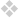# 3

1.
2 is another.
3 is made from 2 and 1.
4 is 3 which is 2 and 1 and all their modalities
5 is the current produced by 4 which is 3 and 2 and 1 and all their modalities.
6 is 1 atop then the other atop then the other then the other then the other.
7 is transcendent.

1 is another.
2 is itself and its inverse.
3 is 1 and its inverse and a mirror.
4 is 1 and its inverse, a mirror and intersecting geometries.
5 is 4 as arrayed in a cube.
6 is 5 in a series.
7 is prime real estate.
8 is endless
9 is 6 reversed.
10 is 1 and another.
3 is a fragment of 8.

1 is another.  A commons as a circle is the center of town.
2. The center of the center, a hole.
3. A hole is a field of possibilities.
4. It is the custom of the inhabitants to gather periodically and try to remember.
5. Because it has long been this way the men wear derbies and overcoats, the women Victorian dresses.
6. They stand together at the edge.
7. Hands folded behind them, they wait.
8. They feel the air bristle with maybes.
9. But nothing ever happens.
10. It is strange the way momentous things disappear.